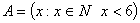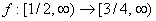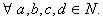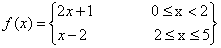Visitors Online: 0 | Saturday 07th December 2019CBSE Guess > Papers > Important Questions > Class XII > 2008 > Maths > Maths By Mrs. Geethanjali Bhatt CBSE CLASS XII
Q. 1. Let A = {1,2,3,4} find the number of relations on A containing (1, 2) and (3, 2) which is reflexive transitive but not symmetric giving sufficient reasons. Q. 2. Let R be the set of real numbers on ‘R’ defined by R = {(a,b) / | a-b | < 5} is not transitive . Prove the above statement by giving two examples. Q. 3.R= { (a,b): |a-b| is even} Find whether the relation R on A is equivalence. All the elements of {1,3,5} is not related to any element of {2,4} with respect to R. True/False . Justify your answer. Q. 4. Let Q be the set of all rational numbers on R be the relation on Q defined by R = { (x,y) : 1 + xy > 0} Find whether relation is equivalence. Q. 5. f : z -> z f(x) = x2 + x. rove that the function is neither injective nor subjective. Q. 6. Letwhere f(x) = x2 – x+ 1.Prove that f is invertible and hence find f -1. Q. 7. Let f: N --> Y be a function defined by f(x) = x2 + 1 Show that f is one – one and replace Y by a set so that f is invertible. Also find its inverse function. Q. 8. Let f : R -> R g: R -> R defined by f(x)=x2 + 8 g(x)=3x3 + 1 f(x)=x2 + 2x – 3 g(x) = 3x – 4 Show that fog and gof exists and hence find them. Q. 9.Let N be the set of all natural numbers. R be the relation on N X N defined by (a,b) R (c,d) iff ad = bcShow that R is equivalence. Q. 10.Q. 11.Is the function one-one onto Q. 12. A function f over the set of real numbers is defined asFind whether the function is one-one or onto